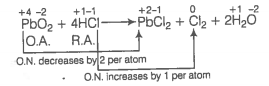PbO and PbO2 react with HCl according to following chemical equations

$2\mathrm{PbO}+4\mathrm{HCl}\to 2{\mathrm{PbCl}}_{2}+2{\mathrm{H}}_{2}\mathrm{O}$

${\mathrm{PbO}}_{2}+4\mathrm{HCl}\to {\mathrm{PbCl}}_{2}+{\mathrm{Cl}}_{2}+2{\mathrm{H}}_{2}\mathrm{O}$

Why do these compounds differ in their reactivity?

Writing the oxidation number of each element above its symbol in the following reactions
(a)
In this reaction, oxidation number of each element remains same hence, it is not a redox reaction. In fact, it is an example of acid-base reaction.
(b) $\stackrel{+4}{\mathrm{Pb}}{\stackrel{-2}{\mathrm{O}}}_{2}+4\stackrel{+1}{\mathrm{H}}\stackrel{-1}{\mathrm{Cl}}\to \stackrel{+2}{\mathrm{Pb}}{\stackrel{-1}{\mathrm{Cl}}}_{2}+{\stackrel{0}{\mathrm{Cl}}}_{2}+2{\stackrel{+1}{\mathrm{H}}}_{2}\stackrel{-2}{\mathrm{O}}$
In PbO2, Pb is in +4 oxidation state. Due to inert pair effect Pb in +2 oxidation state is more stable. So, Pb in +4 oxidation state (PbO2) acts as an oxidising agent.
It oxidises Cl- to Cl2 and itself gets reduced to Pb2+.